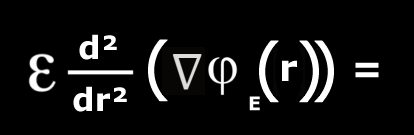# Mystery equation

## Recommended Posts

I am hoping that people here might be able to provide insight into
what context/s this equation might be relevant, particularly the contents of the brackets.

I am aware it is a strange request, related to puzzle solving,
but perhaps someone can help guide me in an interesting direction.##### Share on other sites

The term inside is the gradient of phiE, which is a function of r.

In physics, that could describe the gradient of a potential; the gradient of the electric potential is the electric field (with a minus sign in there somewhere).

You are taking the second derivative of this gradient.

##### Share on other sites

Just logged on ans swansont gor there whiles I was drinking mt tea and thinking about this one.

So I will add a little extra.

grad phi means that we are talking about multiple dimensions, probably 3.

So r will be a probably be the radial dimension of cylindrical or spherical coordinates since many potentials will be symmetrically distributed in shells about the origin.

##### Share on other sites

And $$\varepsilon$$ stands for permittivity of a medium?

Vaguely similar to Poisson's equation in electrostatics $$\varepsilon \nabla^2 \varphi = -\rho,$$ where $$\rho$$ is charge distribution.

I should do the dimensional analysis. But trying to get in a first wild guess, I would try $$-\frac{d}{dr} \rho(r)$$ as the RHS of the mystery equation.

Edited by taeto
##### Share on other sites

The second derivative of a potential is concerned with stability.

##### Share on other sites

And the second derivative of the gradient of a potential? With change in stability?

##### Share on other sites

Since the few stabs taken so far concern either electrostatics or mechanical stability, this could be moved to either a physics section, or to the puzzles.

## Create an account

Register a new account